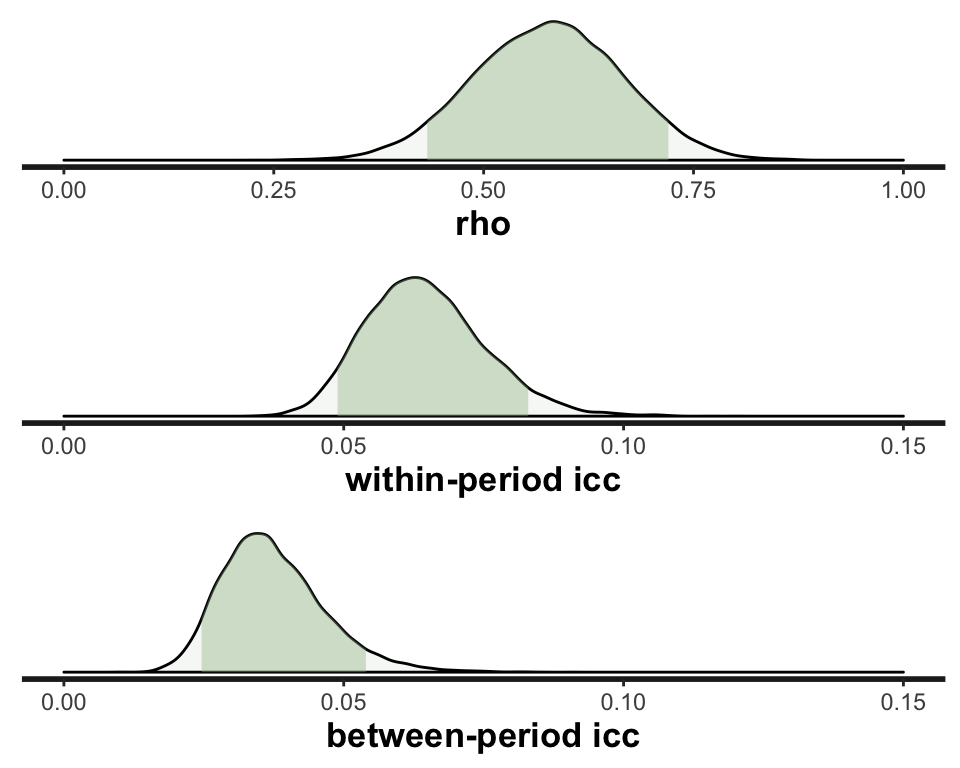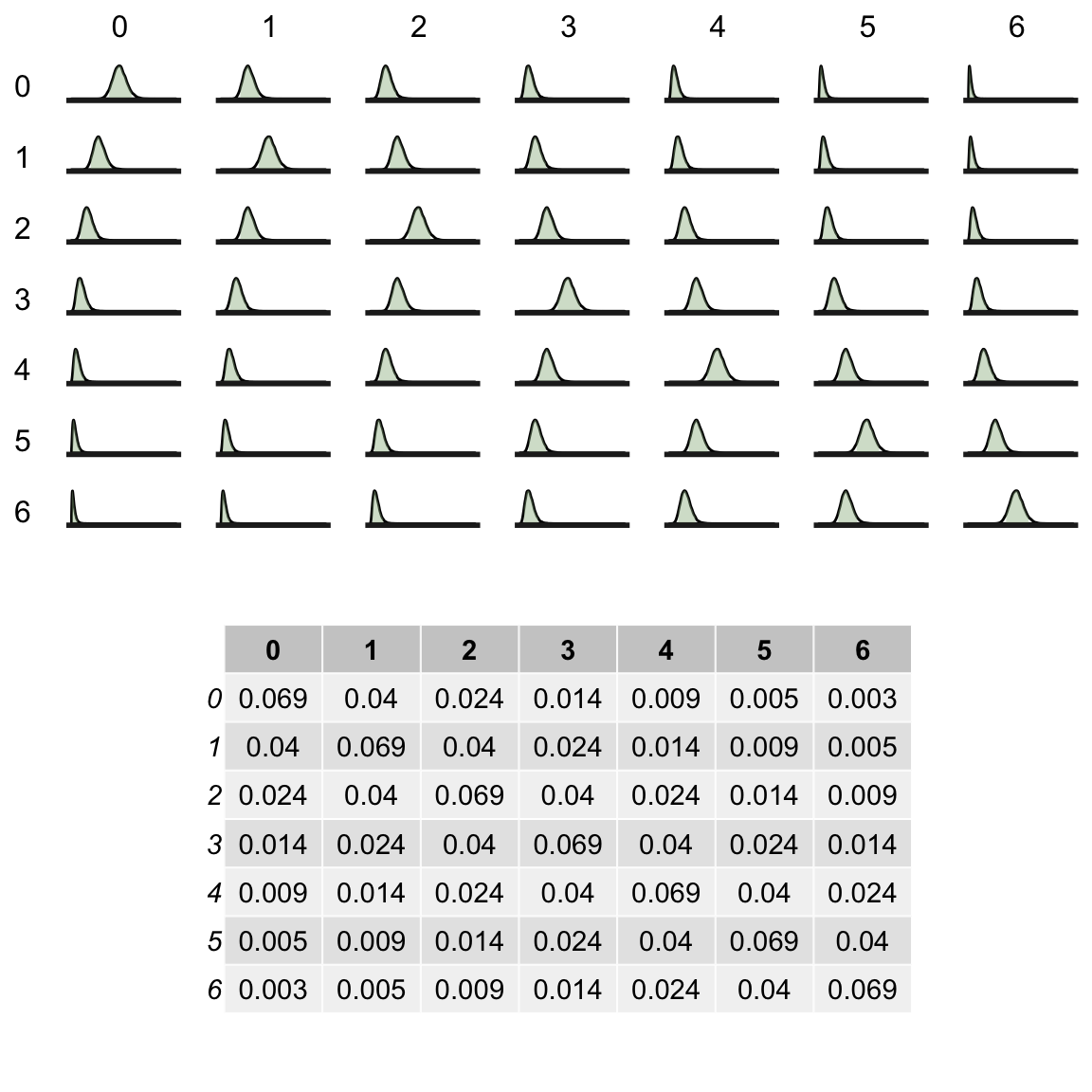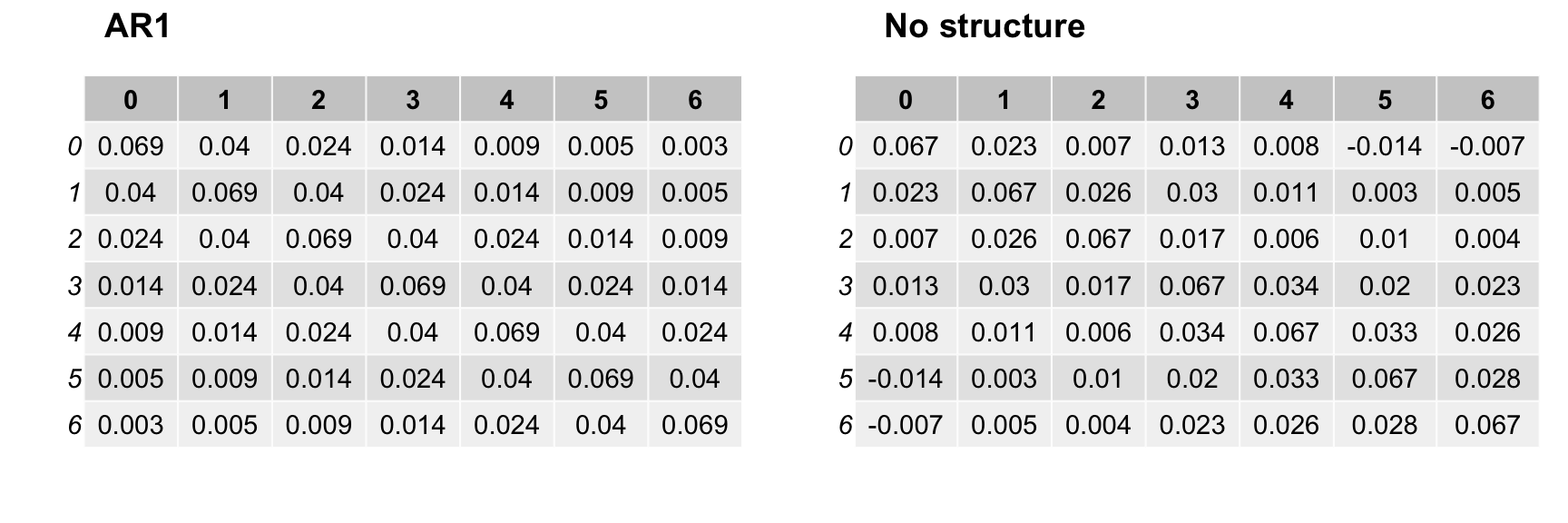# Bayes models for estimation in stepped-wedge trials with non-trivial ICC patterns

Continuing a series of posts discussing the structure of intra-cluster correlations (ICC’s) in the context of a stepped-wedge trial, this latest edition is primarily interested in fitting Bayesian hierarchical models for more complex cases (though I do talk a bit more about the linear mixed effects models). The first two posts in the series focused on generating data to simulate various scenarios; the third post considered linear mixed effects and Bayesian hierarchical models to estimate ICC’s under the simplest scenario of constant between-period ICC’s. Throughout this post, I use code drawn from the previous one; I am not repeating much of it here for brevity’s sake. So, if this is all new, it is probably worth glancing at before continuing on.

### Data generation

The data generating model this time around is only subtly different from before, but that difference is quite important. Rather than a single cluster-specific effect $$b_c$$, there is now a vector of cluster effects $$\mathbf{b_c} = \left( b_{c1}, b_{c2}, \ldots, b_{cT} \right)$$, where $$b_c \sim MVN(\mathbf{0}, \sigma^2 \mathbf{R})$$ (see this earlier post for a description of the correlation matrix $$\mathbf{R}$$.)

$Y_{ict} = \mu + \beta_0t + \beta_1X_{ct} + b_{ct} + e_{ict}$

By altering the correlation structure of $$\mathbf{b_c}$$ (that is $$\mathbf{R}$$), we can the change the structure of the ICC’s. (The data generation was the focus of the first two posts of this series, here and here. The data generating function genDD includes an argument where you can specify the two correlation structures, exchangeable and auto-regressive:

library(simstudy)

defc <- defData(varname = "mu", formula = 0,
dist = "nonrandom", id = "cluster")
defc <- defData(defc, "s2", formula = 0.15, dist = "nonrandom")
defc <- defData(defc, "m", formula = 15, dist = "nonrandom")

formula = "0 + 0.10  * period + 1 * rx + cteffect",
variance = 2, dist = "normal")
genDD <- function(defc, defa, nclust, nperiods,
waves, len, start, rho, corstr) {

dc <- genData(nclust, defc)
dp <- trtStepWedge(dp, "cluster", nWaves = waves, lenWaves = len,
startPer = start)
dp <- addCorGen(dtOld = dp, nvars = nperiods, idvar = "cluster",
rho = rho, corstr = corstr, dist = "normal",
param1 = "mu", param2 = "s2", cnames = "cteffect")

dd <- genCluster(dp, cLevelVar = "timeID", numIndsVar = "m",
level1ID = "id")
dd[]
}

### Constant between-period ICC’s

In this first scenario, the assumption is that the within-period ICC’s are larger than the between-period ICC’s and the between-period ICC’s are constant. This can be generated with random effects that have a correlation matrix with compound symmetry (or is exchangeable). In this case, we will have 60 clusters and 7 time periods:

set.seed(4119)
dcs <- genDD(defc, defa, 60, 7, 4, 1, 2, 0.6, "cs")

# correlation of "unobserved" random effects

round(cor(dcast(dcs[, .SD, keyby = .(cluster, period)],
formula = cluster ~ period, value.var = "cteffect")[, 2:7]), 2)
##      0    1    2    3    4    5
## 0 1.00 0.60 0.49 0.60 0.60 0.51
## 1 0.60 1.00 0.68 0.64 0.62 0.64
## 2 0.49 0.68 1.00 0.58 0.54 0.62
## 3 0.60 0.64 0.58 1.00 0.63 0.66
## 4 0.60 0.62 0.54 0.63 1.00 0.63
## 5 0.51 0.64 0.62 0.66 0.63 1.00

#### Linear mixed-effects model

It is possible to use lmer to correctly estimate the variance components and other parameters that underlie the data generating process used in this case. The cluster-level period-specific effects are specified in the model as “cluster/period”, which indicates that the period effects are nested within the cluster.

library(lme4)

lmerfit <- lmer(Y ~ period + rx + (1 | cluster/period) , data = dcs)
as.data.table(VarCorr(lmerfit))
##               grp        var1 var2       vcov     sdcor
## 1: period:cluster (Intercept) <NA> 0.05827349 0.2413990
## 2:        cluster (Intercept) <NA> 0.07816476 0.2795796
## 3:       Residual        <NA> <NA> 2.02075355 1.4215321

Reading from the vcov column in the lmer output above, we can extract the period:cluster variance ($$\sigma_w^2$$), the cluster variance ($$\sigma^2_v$$), and the residual (individual level) variance ($$\sigma^2_e$$). Using these three variance components, we can estimate the correlation of the cluster level effects ($$\rho$$), the within-period ICC ($$ICC_{tt}$$), and the between-period ICC ($$ICC_{tt^\prime}$$). (See the addendum below for a more detailed description of the derivations.)

#### Correlation ($$\rho$$) of cluster-specific effects over time

In this post, don’t confuse $$\rho$$ with the ICC. $$\rho$$ is the correlation between the cluster-level period-specific random effects. Here I am just showing that it is function of the decomposed variance estimates provided in the lmer output:

$\rho = \frac{\sigma^2_v}{\sigma^2_v + \sigma^2_w}$

vs <- as.data.table(VarCorr(lmerfit))$vcov vs/sum(vs[1:2])  ##  0.5728948 #### Within-period ICC The within-period ICC is the ratio of total cluster variance relative to total variance: $ICC_{tt} = \frac{\sigma^2_v + \sigma^2_w}{\sigma^2_v + \sigma^2_w+\sigma^2_e}$ sum(vs[1:2])/sum(vs) ##  0.06324808 #### Between-period ICC The between-period $$ICC_{tt^\prime}$$ is really just the within-period $$ICC_{tt}$$ adjusted by $$\rho$$ (see the addendum): $ICC_{tt^\prime} = \frac{\sigma^2_v}{\sigma^2_v + \sigma^2_w+\sigma^2_e}$ vs/sum(vs)  ##  0.0362345 #### Bayesian model Now, I’ll fit a Bayesian hierarchical model, as I did earlier with the simplest constant ICC data generation process. The specification of the model in stan in this instance is slightly more involved as the number of parameters has increased. In the simpler case, I only had to estimate a scalar parameter for $$\sigma_b$$ and a single ICC parameter. In this model definition (nested_cor_cs.stan) $$\mathbf{b_c}$$ is a vector so there is a need to specify the variance-covariance matrix $$\sigma^2 \mathbf{R}$$, which has dimensions $$T \times T$$ (defined in the transformed parameters block). There are $$T$$ random effects for each cluster, rather than one. And finally, instead of one ICC value, there are two - the within- and between-period ICC’s (defined in the generated quantities block). data { int<lower=0> N; // number of unique individuals int<lower=1> K; // number of predictors int<lower=1> J; // number of clusters int<lower=0> T; // number of periods int<lower=1,upper=J> jj[N]; // group for individual int<lower=1> tt[N]; // period for individual matrix[N, K] x; // matrix of predctors vector[N] y; // matrix of outcomes } parameters { vector[K] beta; // model fixed effects real<lower=0> sigmalev1; // cluster variance (sd) real<lower=-1,upper=1> rho; // correlation real<lower=0> sigma; // individual level varianc (sd) matrix[J, T] ran; // site level random effects (by period) } transformed parameters{ cov_matrix[T] Sigma; vector[N] yhat; vector[T] mu0; for (t in 1:T) mu0[t] = 0; // Random effects with exchangeable correlation for (j in 1:(T-1)) for (k in (j+1):T) { Sigma[j,k] = pow(sigmalev1,2) * rho; Sigma[k,j] = Sigma[j, k]; } for (i in 1:T) Sigma[i,i] = pow(sigmalev1,2); for (i in 1:N) yhat[i] = x[i]*beta + ran[jj[i], tt[i]]; } model { sigma ~ uniform(0, 10); sigmalev1 ~ uniform(0, 10); rho ~ uniform(-1, 1); for (j in 1:J) ran[j] ~ multi_normal(mu0, Sigma); y ~ normal(yhat, sigma); } generated quantities { real sigma2; real sigmalev12; real iccInPeriod; real iccBetPeriod; sigma2 = pow(sigma, 2); sigmalev12 = pow(sigmalev1, 2); iccInPeriod = sigmalev12/(sigmalev12 + sigma2); iccBetPeriod = iccInPeriod * rho; } Model estimation requires creating the data set (in the form of an R list), compiling the stan model, and then sampling from the posterior to generate distributions of all parameters and generated quantities. I should include conduct a diagnostic review (e.g. to assess convergence), but you’ll have to trust me that everything looked reasonable. library(rstan) options(mc.cores = parallel::detectCores()) x <- as.matrix(dcs[ ,.(1, period, rx)]) K <- ncol(x) N <- dcs[, length(unique(id))] J <- dcs[, length(unique(cluster))] T <- dcs[, length(unique(period))] jj <- dcs[, cluster] tt <- dcs[, period] + 1 y <- dcs[, Y] testdat <- list(N=N, K=K, J=J, T=T, jj=jj, tt=tt, x=x, y=y) rt <- stanc("nested_cor_cs.stan") sm <- stan_model(stanc_ret = rt, verbose=FALSE) fit.cs <- sampling(sm, data=testdat, seed = 32748, iter = 5000, warmup = 1000, control = list(max_treedepth = 15)) Here is a summary of results for $$\rho$$, $$ICC_{tt}$$, and $$ICC_{tt^\prime}$$. I’ve included a comparison of the means of the posterior distributions with the lmer estimates, followed by a more complete (visual) description of the posterior distributions of the Bayesian estimates: mb <- sapply( rstan::extract(fit.cs, pars=c("rho", "iccInPeriod", "iccBetPeriod")), function(x) mean(x) ) cbind(bayesian=round(mb,3), lmer = round(c(vs/sum(vs[1:2]), sum(vs[1:2])/sum(vs), vs/sum(vs)),3) ) ## bayesian lmer ## rho 0.576 0.573 ## iccInPeriod 0.065 0.063 ## iccBetPeriod 0.037 0.036### Decaying between-period ICC over time Now we enter somewhat uncharted territory, since there is no obvious way in R using the lme4 or nlme packages to decompose the variance estimates when the random effects have correlation that decays over time. This is where we might have to rely on a Bayesian approach to do this. (I understand that SAS can accommodate this, but I can’t bring myself to go there.) We start where we pretty much always do - generating the data. Everything is the same, except that the cluster-random effects are correlated over time; we specify a correlation structure of ar1 (auto-regressive). set.seed(4119) dar1 <- genDD(defc, defa, 60, 7, 4, 1, 2, 0.6, "ar1") # correlation of "unobserved" random effects round(cor(dcast(dar1[, .SD, keyby = .(cluster, period)], formula = cluster ~ period, value.var = "cteffect")[, 2:7]), 2) ## 0 1 2 3 4 5 ## 0 1.00 0.60 0.22 0.20 0.18 0.06 ## 1 0.60 1.00 0.64 0.45 0.30 0.23 ## 2 0.22 0.64 1.00 0.61 0.32 0.30 ## 3 0.20 0.45 0.61 1.00 0.61 0.49 ## 4 0.18 0.30 0.32 0.61 1.00 0.69 ## 5 0.06 0.23 0.30 0.49 0.69 1.00 The model file is similar to nested_cor_cs.stan, except that the specifications of the variance-covariance matrix and ICC’s are now a function of $$\rho^{|t^\prime - t|}$$: transformed parameters{ ⋮ for (j in 1:T) for (k in 1:T) Sigma[j,k] = pow(sigmalev1,2) * pow(rho,abs(j-k)); ⋮ } generated quantities { ⋮ for (j in 1:T) for (k in 1:T) icc[j, k] = sigmalev12/(sigmalev12 + sigma2) * pow(rho,abs(j-k)); ⋮ } The stan compilation and sampling code is not shown here - they are the same before. The posterior distribution of $$\rho$$ is similar to what we saw previously. print(fit.ar1, pars=c("rho")) ## Inference for Stan model: nested_cor_ar1. ## 4 chains, each with iter=5000; warmup=1000; thin=1; ## post-warmup draws per chain=4000, total post-warmup draws=16000. ## ## mean se_mean sd 2.5% 25% 50% 75% 97.5% n_eff Rhat ## rho 0.58 0 0.08 0.41 0.53 0.58 0.64 0.73 2302 1 ## ## Samples were drawn using NUTS(diag_e) at Fri Jun 28 14:13:41 2019. ## For each parameter, n_eff is a crude measure of effective sample size, ## and Rhat is the potential scale reduction factor on split chains (at ## convergence, Rhat=1). Now, however, we have to consider a full range of ICC estimates. Here is a plot of the posterior distribution of all ICC’s with the means of each posterior directly below. The diagonal represents the within-period (constant) ICCs, and the off-diagonals are the between-period ICC’s.### An alternative Bayesian model: unstructured correlation Now, there is no particular reason to expect that the particular decay model (with an AR1 structure) would be the best model. We could try to fit an even more general model, one with minimal structure. For example if we put no restrictions on the correlation matrix $$\mathbf{R}$$, but assumed a constant variance of $$\sigma_b^2$$, we might achieve a better model fit. (We could go even further and relax the assumption that the variance across time changes as well, but I’ll leave that to you if you want to try it.) In this case, we need to define $$\mathbf{R}$$ and specify a prior distribution (I use the Lewandowski, Kurowicka, and Joe - LKJ prior, as suggested by Stan documentation) and define the ICC’s in terms of $$\mathbf{R}$$. Here are the relevant snippets of the stan model (everything else is the same as before): parameters { ⋮ corr_matrix[T] R; // correlation matrix ⋮ } transformed parameters{ ⋮ Sigma = pow(sigmalev1,2) * R; ⋮ } model { ⋮ R ~ lkj_corr(1); // LKJ prior on the correlation matrix ⋮ } generated quantities { ⋮ for (j in 1:T) for (k in 1:T) icc[j, k] = sigmalev12/(sigmalev12 + sigma2) * R[j, k]; ⋮ } Here are the means of the ICC posterior distributions alongside the means from the previous auto-regressive model.Looking at the unstructured model estimates on the right, it does appear that a decay model might be reasonable. (No surprise there, because in reality, it is totally reasonable; that’s how we generated the data.) We can use package bridgesampling which estimates marginal log likelihoods (across the prior distributions of the parameters). The marginal likelihoods are used in calculating the Bayes Factor, which is the basis for comparing two competing models. Here, the log-likelihood is reported. If the unstructured model is indeed an improvement (and it could very well be, because it has more parameters), the we would expect the marginal log-likelihood for the second model to be greater (less negative) than the log-likelihood for the auto-regressive model. If fact, the opposite true, suggesting the auto-regressive model is the preferred one (out of these two): library(bridgesampling) bridge_sampler(fit.ar1, silent = TRUE) ## Bridge sampling estimate of the log marginal likelihood: -5132.277 ## Estimate obtained in 6 iteration(s) via method "normal" bridge_sampler(fit.ar1.nc, silent = TRUE) ## Bridge sampling estimate of the log marginal likelihood: -5137.081 ## Estimate obtained in 269 iteration(s) via method "normal". ## Addendum - interpreting lmer variance estimates In order to show how the lmer variance estimates relate to the theoretical variances and correlations in the case of a constant between-period ICC, here is a simulation based on 1000 clusters. The key parameters are $$\sigma^2_b = 0.15$$, $$\sigma^2_e = 2$$, and $$\rho = 0.6$$. And based on these values, the theoretical ICC’s are: $$ICC_{within} = 0.15/2.15 = 0.698$$, and $$ICC_{bewteen} = 0.698 * 0.6 = 0.042$$. set.seed(4119) dcs <- genDD(defc, defa, 1000, 7, 4, 1, 2, 0.6, "cs") The underlying correlation matrix of the cluster-level effects is what we would expect: round(cor(dcast(dcs[, .SD, keyby = .(cluster, period)], formula = cluster ~ period, value.var = "cteffect")[, 2:7]), 2) ## 0 1 2 3 4 5 ## 0 1.00 0.59 0.59 0.61 0.61 0.61 ## 1 0.59 1.00 0.61 0.60 0.61 0.64 ## 2 0.59 0.61 1.00 0.59 0.61 0.61 ## 3 0.61 0.60 0.59 1.00 0.59 0.62 ## 4 0.61 0.61 0.61 0.59 1.00 0.60 ## 5 0.61 0.64 0.61 0.62 0.60 1.00 Here are the variance estimates from the mixed-effects model: lmerfit <- lmer(Y ~ period + rx + (1 | cluster/period) , data = dcs) as.data.table(VarCorr(lmerfit)) ## grp var1 var2 vcov sdcor ## 1: period:cluster (Intercept) <NA> 0.05779349 0.2404028 ## 2: cluster (Intercept) <NA> 0.09143749 0.3023863 ## 3: Residual <NA> <NA> 1.98894356 1.4102991 The way lmer implements the nested random effects , the cluster period-specific effect $$b_{ct}$$ is decomposed into $$v_c$$, a cluster level effect, and $$w_{ct}$$, a cluster time-specific effect: $b_{ct} = v_c + w_{ct}$ Since both $$v_c$$ and $$w_{ct}$$ are normally distributed ($$v_c \sim N(0,\sigma_v^2)$$ and $$w_{ct} \sim N(0,\sigma_w^2)$$), $$var(b_{ct}) = \sigma^2_b = \sigma^2_v + \sigma^2_w$$. Here is the observed estimate of $$\sigma^2_v + \sigma^2_w$$: vs <- as.data.table(VarCorr(lmerfit))$vcov
sum(vs[1:2])
##  0.149231

An estimate of $$\rho$$ can be extracted from the lmer model variance estimates:

\begin{aligned} \rho &= cov(b_{ct}, b_{ct^\prime}) \\ &= cov(v_{c} + w_{ct}, v_{c} + w_{ct^\prime}) \\ &= var(v_c) + cov(w_{ct}) \\ &= \sigma^2_v \end{aligned}

\begin{aligned} var(b_{ct}) &= var(v_{c}) + var(w_{ct}) \\ &= \sigma^2_v + \sigma^2_w \end{aligned}

\begin{aligned} cor(b_{ct}, b_{ct^\prime}) &= \frac{cov(b_{ct}, b_{ct^\prime})}{\sqrt{var(b_{ct}) var(b_{ct^\prime})} } \\ \rho &= \frac{\sigma^2_v}{\sigma^2_v + \sigma^2_w} \end{aligned}

vs/sum(vs[1:2])
##  0.6127246

And here are the estimates of within and between-period ICC’s:

$ICC_{tt} = \frac{\sigma^2_b}{\sigma^2_b+\sigma^2_e} =\frac{\sigma^2_v + \sigma^2_w}{\sigma^2_v + \sigma^2_w+\sigma^2_e}$

sum(vs[1:2])/sum(vs)
##  0.06979364

\begin{aligned} ICC_{tt^\prime} &= \left( \frac{\sigma^2_b}{\sigma^2_b+\sigma^2_e}\right) \rho \\ \\ &= \left( \frac{\sigma^2_v + \sigma^2_w}{\sigma^2_v + \sigma^2_w+\sigma^2_e}\right) \rho \\ \\ &=\left( \frac{\sigma^2_v + \sigma^2_w}{\sigma^2_v + \sigma^2_w+\sigma^2_e} \right) \left( \frac{\sigma^2_v}{\sigma^2_v + \sigma^2_w} \right) \\ \\ &= \frac{\sigma^2_v}{\sigma^2_v + \sigma^2_w+\sigma^2_e} \end{aligned}

vs/sum(vs)
##  0.04276428